# Quartz Crystal Oscillators Electrical Engineering (EE) Notes | EduRev

## Electrical Engineering (EE) : Quartz Crystal Oscillators Electrical Engineering (EE) Notes | EduRev

The document Quartz Crystal Oscillators Electrical Engineering (EE) Notes | EduRev is a part of the Electrical Engineering (EE) Course Electronic Devices.
All you need of Electrical Engineering (EE) at this link: Electrical Engineering (EE)

Quartz Crystal Oscillators

One of the most important features of an y oscillator is its frequency stabilit y, or in  other words its ability to provide a constant frequenc y   output under varying load conditions. Some of the factors that affect the frequency stability of an oscillator include: temperature, variations in the load and changes in the DC power supply.

Frequency stabilit y of the output signal can be   improved by the proper selection of the components used for the resonant feedback circuit including the amplifier but there is a limit to the stability that can be obtained from normal LC and RC tank circuits.
To obtain a ver y high  level of oscillator stability a Quartz Crystalis  generall y used as the frequency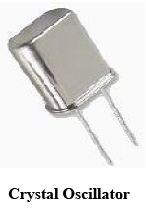When a voltage  source is applied to a  small thin piece of quartz crystal, it begins to change shape producing a characteristic known as the Piezo- electric effect.

This piezo- electric effect is the  propert y of a crystal b y which an electrical charge produces a mechanical force b y changing the shape of the crystal and vice versa, a mechanical force applied to the crystal produces an electrical charge.

Then, piezo- electric devices can be classed as Transducersas they convert energy of one kind into energy of another (electrical to mechanical or mechanical to electrical).

This piezo- electric effect produces mechanical vibrations or oscillations which are used to replace the LC tank circuit in the previous oscillators.

There are many different types of crystal substances which can be used as oscillators with the most important of these for electronic circuits being the quartz minerals because  of their greater mechanical strength.

The quartz crystal used in a Quartz Crystal Oscillator is a very small, thin piece or wafer of cut quartz with the two parallel surfaces metallised to make the required electrical connections. The physical size and thickness of a piece of quartz crystal is tightl y controlled since it affects the final frequency of oscillations and is called the crystals "characteristic frequency". Then once cut and shaped, the crystal can not be used at an y other frequenc y. In other words, its size and shape determines its frequency.

Quartz Crystal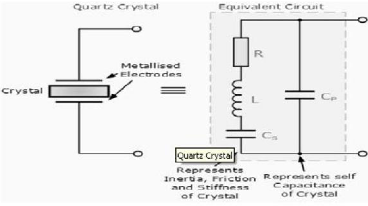The equivalent circuit for the quartz crystal shows an RLC series circuit, which represents the mechanical vibrations of the crystal, in parallel with a capacitance, Cp which represents the electrical connections to  the cr ystal. Quartz crystal oscillators operate at "parallel resonance", and the equivalent impedance of the crystal has a series resonance where Cs resonates with inductance, L and a parallel resonance where L resonates with the series combination of Cs and Cp as shown.

Crystal Reactance : The slope of the reactance against frequenc y above, shows that the series reactance at frequency ƒs is inversel y proportional to Cs because below  ƒs and above ƒp the crystal appears capacitive, i.e. dX/dƒ, where X is the reactance.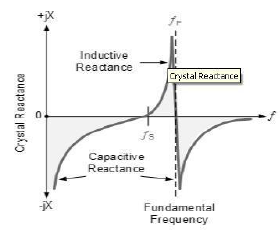The slope of the reactance against frequency above, shows that the series reactance at frequency fs is inversely proportional to Cs becaus e below fs and above fp the crystal appears capacitive, i.e. dX/d f, where X is the reactance. Between frequencies ƒs and ƒp , the crystal app ears inductive as the two parallel capacitances cancel out. The point where the reactance values of the capacitances and inductance cancel each other out Xc = XL is the fundamental frequenc y of the crystal.

A quartz cr ystal has a resonant frequency similar to that of a electricall y tuned tank circuit butwith a much higher Q factor due to its low resistance, with typical frequencies ranging from 4kHz to 10MHz. The cut of the crystal also determines how it will behave as some crystals will vibrate at more than one frequency. Also, if the cr ystal is not of a paral lel or  uniform thickness it has two or more resonant frequencies having both a fundamental frequency and harmonics such as second or third harmonics. However, usually the fundamental frequency is more stronger or pronounced than the others and this is the one used. The equivalent circuit above has three reactive components and there are two resonant frequencies, the lowest is a series type frequenc y and the highest  a parallel type resonant frequenc y.

We have seen in the previous tutorials, that an amplifier circuit will oscillate if it has  a loop gain greater or equal to one and the feedback is positive. In a Quartz Crystal Oscillator circuit the oscillator will oscillate at the crystals fundamental parallel resonant frequency as the cr ystal alwa ys wants to oscillate when a voltage source is applied to it.

Colpitts Crystal Oscillator:

The design of a Crystal Oscillator is very similar to the design of the Colpitts Oscillator we looked at in the previous tutorial, except that the LC tank circuit has been replaced b y a quartz crystal as shown below.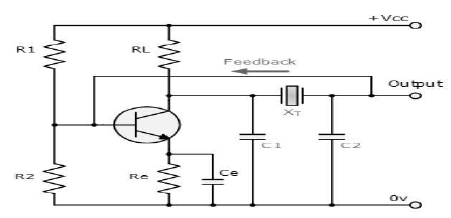These types of Crystal Oscillators are designed around the common emitter amplifier stage of a Colpitts Oscillator. The input signal to the base of the transistor is inverted at the transistors output. The output signal at the collector is then taken through a 180o phase  shifting network which includes the crystal operating in a series resonant mode. The output is also fed back to the input which is "in- phase" with the input providing the necessary positive feedback. Resistors, R1 and R2 bias the resistor in aClass A type operation while resistor Re is chosen so that the loop gain is slightl y greater than unit y.

Capacitors, C1 and C2 are made as large as possible   in order that the frequency of oscillations can approximate to the series resonant mode of the crystal and  is  not dependant upon the values of these capacitors. The circuit diagram above of the Colpitts Crystal Oscillator circuit shows that capacitors, C1 and C2 shunt the output of the transistor which reduces the feedback signal. Therefore, the gain of the transistor limits the maximum values of C1 and C2.

The output amplitude should be kept low in order to avoid excessive power dissipation in the crystal otherwise could destroy itself by excessive vibration.

Pierce Oscillator

The Pierce oscillator is a cr ystal oscillator that uses the cr ystal as part of its feedback path and therefore has no resonant tank circuit. The Pierce Oscillator uses a JFET as its amplifying device as it provides a very high input impedance  with the crystal connected between the  output Drain terminal and the input Gate terminal as shown below.

Pierce Crystal Oscillator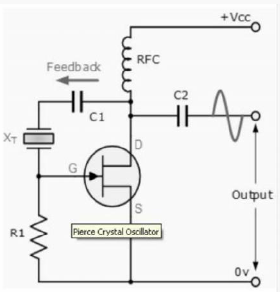In this simple circuit, the crystal determines the frequency of oscillations and operates    on its series resonant frequency giving a low impedance path between output and input.

There is a 180° phase shift at resonance, making  the feedback positive. The amplitude of the output sine wave is limited to the maximum voltage range at the Drain terminal.

Resistor, R1 controls the amount of feedback and crystal drive while the voltage across the radio frequency choke, RFC reverses during each cycle. Most digital clocks, watches and timers use a Pierce  Oscillator in some form or other as it can be implemented using the minimum of components.

Offer running on EduRev: Apply code STAYHOME200 to get INR 200 off on our premium plan EduRev Infinity!

## Electronic Devices

53 videos|56 docs|97 tests

,

,

,

,

,

,

,

,

,

,

,

,

,

,

,

,

,

,

,

,

,

;